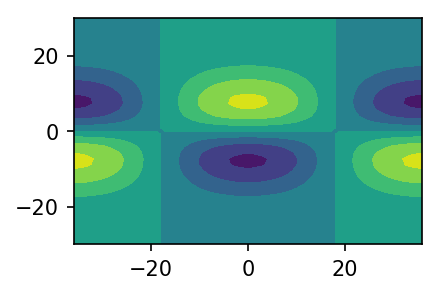Matsuno Analytical Wave Solution implemented in Python

## pymaws: Matsuno Analytical Wave Solution implemented in Python

A python module for evaluating the initial conditions used in: The Matsuno baroclinic wave test case (under review for GEOSCI. MODEL DEV.).

### Installation

pymaws has minimal requirements of:

• Python 3.4 to 3.7
• numpy 1.16

The package can be installed using pip:

$pip install pymaws  ### Testing The testing procedure tests all the parts of pymaws and should take anywhere from a few seconds upto 20 seconds due to random elements in the tests. To run the tests, use python3 in the command line: $ python test_pymaws.py


You should get OK in the last line.

### Getting Started

In the python environmnet, start by importing pymaws with the command:

$from pymaws import *  The main function eval_field was loaded to your environment and a dictionary named Earth that stores the planetary parameters used in this package. (if you want to run eval_field with different parameters , see below) ### Example Let's begin with a regular grid of lat/lon on a 20 second time interval: $ import numpy as np
$nlats = 100$ nlons = 200
$ntime = 50$ lats = np.deg2rad(np.linspace(-80, 80, nlats))
$lons = np.deg2rad(np.linspace(-180, 180, nlons))$ time = np.linspace(0.0, 20, ntime)


Now, let's evaluate the meridional velocity field of an Eastward propagating Inertia-Gravity (EIG) wave:

$v = np.zeros((ntime, nlats, nlons))$ for t in range(ntime):
$for j in range(nlats):$         for i in range(nlons):
$v[t, j, i] = eval_field(lats[j], lons[i], time[t],$                                           field='v', wave_type='EIG')

$v.shape$ (50, 200, 100)


Note that the default arguments of eval_field are n=1, k=5, amp=1e-5, wave_type='Rossby' and parameters=Earth. This package does not include visualizations of any kind, but you can use matplotlib, e.g.

$from matplotlib import pyplot as plt$ plt.contourf(np.rad2deg(lons), np.rad2deg(lats), v[0, :, :])
$plt.xlim(-36,36)$ plt.ylim(-30,30)Meridional velocity at t=0

### Caveats

This version of pymaws does not solve Matsuno equations for n, k < 1,

### Planetary Parameters:

The default parameters in pymaws are stored in a dictionary named Earth:

${'angular_frequency': 7.29212e-05, 'gravitational_acceleration': 9.80616, 'mean_radius': 6371220.0, 'layer_mean_depth': 30.0}  If you want to use different planetary parameters, just copy the dictionary Earth and replace the appropriate values. For example lets change the layer_mean_depth parameter to 10 meters: $   Earth_1 = Earth.copy()
\$   Earth_1['layer_mean_depth'] = 10.0


When you run eval_field, remember to use the argument parameters=Earth_1.

### How to cite pymaws

If you use pymaws in your academic work and publish a paper, we kindly ask that you cite pymaws using the following DOI:

## Project details

This version0.1.0

Uploaded source
Uploaded py3